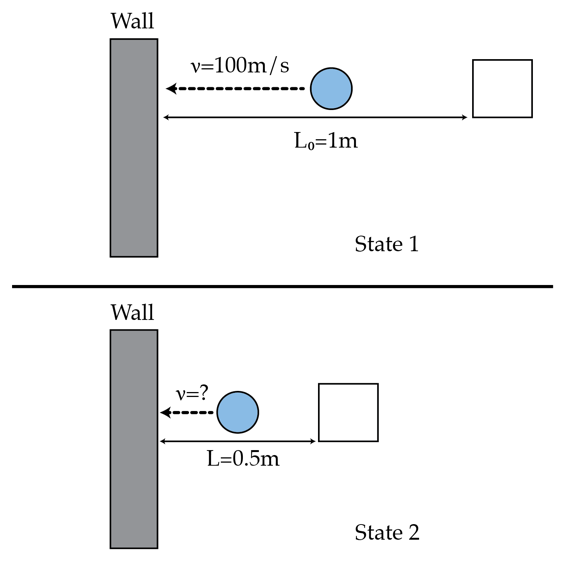# Boinga, boinga, boinga goes the ball

Sometime, even with only a very slow motion, you can still create a big change in a classical mechanics system. Consider the motion in 1 dimension of a ball between a wall (which is fixed) and a block (which is moveable). The distance between the block and the wall is $L_o=1~m$ and the speed of the ball is very large $v_o=100~m/s$. The ball collides perfectly elastically with the block and the wall.

Let's move the block slowly to reduce the distance between it and the wall. Estimate the speed of the ball in meters-per-second when the distance is $L=0.5~m$Details and assumptions

Neglect the size of the ball

×